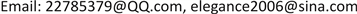1海军驻哈尔滨七O三所军事代表室，黑龙江 哈尔滨

2海军工程大学 动力工程学院，湖北 武汉1. 引言

2. 仿真分析框架

3. 系统建模3.1. 船舶任务模型

3.2. 维修系统模型

3.3. 装备系统模型

4. 可靠性分析流程

4.1. 检查状态转移

4.2. 产生下一批次事件到达时间

4.3. 工作和预测调整

1) 当一批维修保障资源到达后，故障维修过程仿真模块根据新资源安排修理工作，并根据重新安排的修理工作开始修理时间和保障资源计算；如果修理工作消耗的保障资源超过库存则计算保障资源的补充量，保障资源筹供仿真模块根据新的补充需求产生补充时间并把最小补充时间提交给系统调度模块。

2) 当修理工作完成后，触发船舶使用仿真模块，使用模块判断是否改变系统的工作状态并预测最小故障时间；同时故障维修过程仿真模块检查修理队列并重新安排修理工作，并计算修理时间；修理工作可能导致维修保障资源消耗，所以须检查库存，根据存储策略判断是否触发保障资源筹供仿真模块。

3) 当故障发生时，直接触发船舶使用仿真模块，重新调整系统工作状态，流程与(2)相同。

5. 案例研究5.1. 任务描述

5.2. 最大航速及油耗

V = V 0 Q f / Q f 0 3 ， V = V 0 η / η 0 3 (2)

5.3. 任务可靠性仿真结果

5.4. 装备性能退化的影响分析

1) 锅炉上水量下降

2) 系统效率下降

Relationship of power and fuel consumption rate under different spee

Probability of success under mission phase

Probability of success in Navigation and return phase under different required time and feed wate

80 (h)Pn = 100%，Pr = 100% Pn = 100%，Pr = 100%Pn = 100%，Pr = 100%
70 (h)Pn = 98.4%，Pr = 100%Pn = 97.7%，Pr = 100%Pn = 83.5%，Pr = 93.8%
60 (h)Pn = 86.1%，Pr = 92.3%Pn = 97.1%，Pr = 100%Pn = 81.2%，Pr = 94.6%

Probability of success in Navigation starts and return phase under different required time and efficienc

Qf = 0.9Qf0，kt = 0.95kt0Pn = 100%，Pr = 100%， Pq = 80.1%Pn = 98.4%，Pr = 100%， Pq = 80.1%，Pn = 86.1%，Pr = 92.3%， Pq = 80.1%
Qf = 0.8Qf0，kt = 0.9kt0Pn = 100%，Pr = 100%， Pq = 80.9%Pn = 97.7%，Pr = 100%， Pq = 80.9%Pn = 83.5%，Pr = 93.8%， Pq = 80.9%
Qf = 0.7Qf0 kt = 0.85kt0Pn = 100%，Pr = 100%， Pq = 82.2%Pn = 97.1%，Pr = 100%， Pq = 82.2%Pn = 81.2%，Pr = 94.6%， Pq = 82.2%

6. 结束语